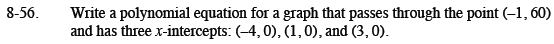Home > CCA2 > Chapter 8 > Lesson 8.1.3 > Problem8-56

8-56.

Write a polynomial equation for a graph that passes through the point (−1, 60) and has three x-intercepts: (−4, 0), (1, 0), and (3, 0). Homework Help ✎Start by writing an equation with those intercepts using the Zero Product Property.
p(x) = a(x + 4)(x − 1)(x − 3)

Find the stretch factor by substituting the point.